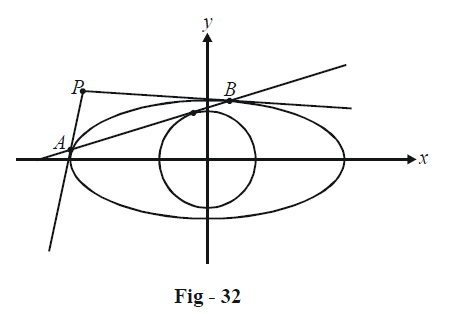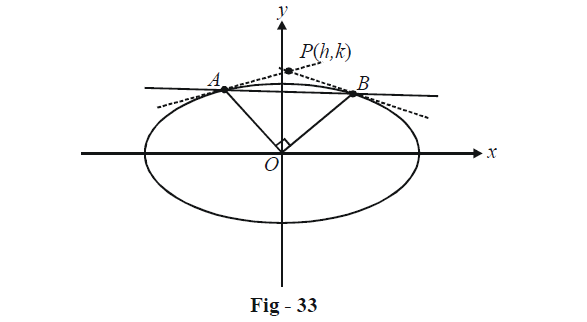# Examples On Tangents And Chords Of Ellipses Set-1

Go back to  'Ellipse'

Example - 26

Find the locus of the point such that the chord of contact of the tangents drawn from it to the ellipse $$\frac{{{x^2}}}{{{a^2}}} + \frac{{{y^2}}}{{{b^2}}} = 1$$ touches the circle $${x^2} + {y^2} = {r^2}.$$

Solution: Let $$P(h,\,k)$$ be such a point.The equation of the chord of contact AB from P(h, k) is

\begin{align}&T(h,\,\,k) = 0\\ & \Rightarrow\quad\frac{{hx}}{{{a^2}}} + \frac{{ky}}{{{b^2}}} = 1\\ & \Rightarrow\quad y = \left( {\frac{{ - {b^2}h}}{{{a^2}k}}} \right)x + \frac{{{b^2}}}{k}\end{align}

This is a tangent to the circle $${x^2} + {y^2} = {r^2},$$ if the condition for tangency for the case of circles $$({c^2} = {a^2}(1 + {m^2}))$$ is satisfied :

\begin{align} &\qquad \frac{{{b}^{4}}}{{{k}^{2}}}={{r}^{2}}\left( 1+\frac{{{b}^{4}}{{h}^{2}}}{{{a}^{4}}{{k}^{2}}} \right)\\ & \Rightarrow \quad {{a}^{4}}{{b}^{4}}={{a}^{4}}{{r}^{2}}{{k}^{2}}+{{b}^{4}}{{r}^{2}}{{h}^{2}} \\ & \Rightarrow \quad \frac{{{h}^{2}}}{{{\left( {}^{{{a}^{2}}}\!\!\diagup\!\!{}_{r}\; \right)}^{2}}}+\frac{{{k}^{2}}}{{{\left( {}^{{{b}^{2}}}\!\!\diagup\!\!{}_{r}\; \right)}^{2}}}=1 \\ \end{align}

Thus, the locus of P is

\begin{align}\frac{{{x}^{2}}}{{{\left({}^{{{a}^{2}}}\!\!\diagup\!\!{}_{r}\;\right)}^{2}}}+\frac{{{y}^{2}}}{{{\left({}^{{{b}^{2}}}\!\!\diagup\!\!{}_{r}\; \right)}^{2}}}=1\end{align}

which is an ellipse

Example - 27

A variable chord AB of the ellipse \begin{align}\frac{{{x^2}}}{{{a^2}}} + \frac{{{y^2}}}{{{b^2}}} = 1\end{align} subtends a right angle at its centre. Tangents drawn at A and B intersect at P. Find the locus of P.

Solution: Let P be the point (h, k).Since AB is the chord of contact for the tangents drawn form P, the equation of AB will be

\begin{align}&T(h,\,k) = 0\\ &\Rightarrow \quad \frac{{hx}}{{{a^2}}} + \frac{{ky}}{{{b^2}}} = 1...\left( 1 \right)\end{align}

We can now write the joint equation of OA and OB by homogenizing the equation of the ellipse using the equation of the chord AB obtained in (1) :

Joint equation of AB :\begin{align}\frac{{{x^2}}}{{{a^2}}} + \frac{{{y^2}}}{{{b^2}}} = {\left( {\frac{{hx}}{{{a^2}}} + \frac{{ky}}{{{b^2}}}} \right)^2}\quad\quad\quad...\left( 2 \right)\end{align}

Since OA and OB are perpendicular, we must have

${\rm{Coeff}}{\rm{. of \;}}{x^2} + {\rm{Coeff}}{\rm{. of\; }}{y^2} = 0{\rm{ }}\quad\quad in{\rm{ }}\left( 2 \right)$

\begin{align}& \Rightarrow \quad \frac{1}{{{a^2}}} - \frac{{{h^2}}}{{{a^4}}} + \frac{1}{{{b^2}}} - \frac{{{k^2}}}{{{b^4}}} = 0\\& \Rightarrow\quad \frac{{{h^2}}}{{{a^4}}} + \frac{{{k^2}}}{{{b^4}}} = \frac{1}{{{a^2}}} + \frac{1}{{{b^2}}}\end{align}

The locus of P is therefore

$\frac{{{x^2}}}{{{a^4}}} + \frac{{{y^2}}}{{{b^4}}} = \frac{1}{{{a^2}}} + \frac{1}{{{b^2}}}$